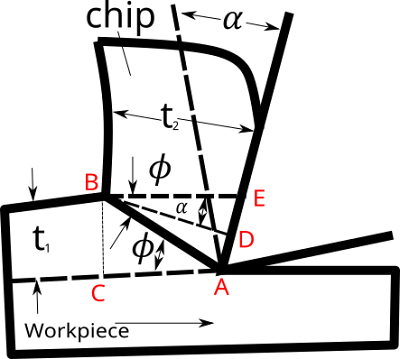# What is Shear Plane Angle in machining and its derivation

What is Shear Plane Angle in machining and its formula derivation

What is Shear Plane Angle in Tool Machining?

It is defined as the plane angle at which the chip is start separating from the workpiece during the metal cutting operation.

With the help shear angle you can calculate cutting force , friction force , shear force, surface smoothness and efficiency of metal removal process

Derivation of Shear angle in machining calculationGeometry of Orthogonal chip formation

Assumption

Let shear plane angle is denoted by \phi

Let Rake angle is denoted by  \alpha

Let thickness of chip before cutting is t_{1}

Let thickness of chip after cutting is t_{2}

Let chip thickness ratio (chip thickness before cutting /chip thickness after cutting) =r_{c}

from the figure we can see right angled triangle ABC

\frac{BC}{AB} = sin \phi

= AB = \frac{BC}{sin \phi } =   \frac{ t_{1} }{sin \phi}    ........(1)

From right angled triangel ABD,

\frac{BD}{AB}  = sin(90- \phi - \alpha ) = cos( \phi - \alpha )

 \frac{ t_{2}}{AB}  =cos( \phi - \alpha )    .......(2)

From equation (1) and (2) , we get

\frac{ t_{1} }{sin \phi } =  \frac{ t_{2} }{cos( \phi - \alpha )}

put the value of  t_{1} and t2 in chip thickness raito

= r_{c} =  \frac{t_{1}}{t_{2}}  =  \frac{sin \phi }{cos( \phi - \alpha )} =  \frac{sin \phi}{cos \phi cos \alpha +sin \phi sin \alpha}

= \frac{r_{c}cos \phi cos \alpha}{sin \phi } + \frac{r_{c}sin \phi sin \alpha}{sin \phi} =1

= \frac{r_{c}cos \alpha}{tan\phi } + r_{c} sin \alpha =1

= \frac{r_{c}cos \alpha}{tan\phi } =1- r_{c} sin \alpha

= tan \phi  =  \frac{ r_{c} cos  \alpha  }{1- r_{c} sin \alpha }